Next: About this document ... Up: lab_template Previous: lab_template

Subsections

# MA 1024 Lab 5: Finding Global Extrema

## Purpose

The purpose of this lab is to acquaint you with techniques for finding global extreme values of functions of two variables.

### Locating Global Extrema

In one-dimensional calculus, the absolute or global extreme values of a function occur either at a point where the derivative is zero, a boundary point, or where the derivative fails to exist. The situation for a function of two variables is very similar, but the problem is more complicated because the boundary now consists of curves instead of just endpoints of intervals. For example, suppose that we wanted to find the global extreme values of a function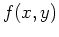on the rectangle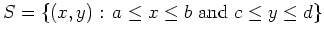. The boundary of this rectangle consists of the four line segments given below.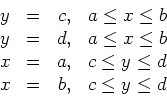We know that the global extrema occur either inside the three-dimensional domain where the derivative is zero or doesn't exist, where the two-dimensional boundaries equal zero or doesn't exist, or at one of the corners.

## Maple Commands

In order to find the absolute (or global) extrema given the paraboloid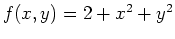whose domain is the rectangle made of the four lines, first enter the three-dimensional function.
>f:=(x,y)->2+x^2+y^2;

Keep your work organized; you may want to work your way through dimensions. Starting with the three dimensional function find the critical points by setting both partials equal to zero; you can do this in one command line. (Notice that there are no undefined points)
>solve({diff(f(x,y),x)=0,diff(f(x,y),y)=0},{x,y});

Next find the critical points along the two-dimensional domain which is the rectangular boundary.The trick is to replace one of the variables with part of the boundary.Since there are four 2-d functions (or sides) this will be done four times.
>solve(diff(f(-1,y),y)=0);
>solve(diff(f(3,y),y)=0);
>solve(diff(f(x,-1),x)=0);
>solve(diff(f(x,3),x)=0);

This gives us the four points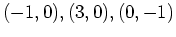, and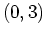. Now the one-dimensional domain is simply the corners: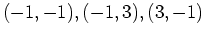, and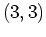. Now that you have all possible points listed you simply need to plug them into the original function to find the nine z-values. The highest and lowest will be the absolute maximum and absolute minimum.

## Exercises

1. Consider the function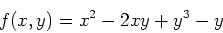Find the absolute extrema of this function on the rectangle,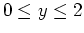.

2. Find the global extrema for the function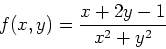on the circular regiongiven by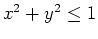.Next: About this document ... Up: lab_template Previous: lab_template
Jane E Bouchard
2009-11-09